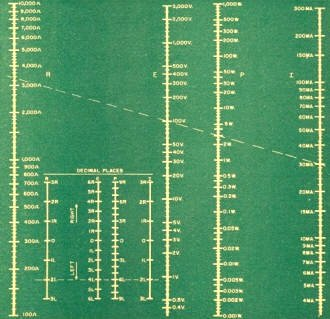# E, I R, and P ChartDecember 1954 Popular Electronics

 December 1954 Popular ElectronicsTable of Contents Wax nostalgic about and learn from the history of early electronics. See articles from Popular Electronics, published October 1954 - April 1985. All copyrights are hereby acknowledged.

Maybe given the nature of newly published Popular Electronics, the editors decided using a big word like "nomograph" might be a little too out of the realm for use in a magazine seeking to appeal to newcomers to the electronics field. It is a little surprising since students of the day were quite accustomed to using this type of a graph since computers still filled entire rooms and hand-held calculators went by a different name - slide rules.

In fact, because of a familiarity with using a slide rule, people were more accustomed to having to shift decimal points to the left or right first to do the calculation on a device that only displayed values in a single decade range, and then to arrive at the final answer after the calculation. That is exactly the skill needed to use the nomograph.

I guess that people today - even engineers - would have a harder time keeping track of powers of 10 than most reasonably skilled high school math students of the 1950s.

E, I R, and P Chart

IF YOU know any two of the four quantities. voltage (E), current (I), resistance, (R). and power (P), in a circuit, you can find the other two by using this chart. Using the upper part of the chart. just lay a straightedge between the graduations corresponding to the two quantities you know, then read the other two quantities where the straight­edge crosses the corresponding lines.Voltage, Current, Resistance, and Power Nomograph

For example, suppose the voltage is 100 volts and current is 30 milliamperes. The resistance is 3330 ohms (approximately) and the power is 3.0 walls.

If the quantities you have are larger or smaller than any shown in the chart, you still can find your answer. Move the decimal point in each quantity to bring it within the range of the chart. In a power figure. move the decimal point a multiple of three places; in a voltage figure, a multiple of two places. In current and resistance, the decimal point can be moved any number of places. Then, using the lower part of the chart, lay your straightedge between the numbers corresponding to the number of places you must move the decimal point on the chart left or right to get the quantities you actually have. Read along the straightedge the number of places you will have to move the decimal point in each answer as given by the chart, to get the proper decimal point for your actual problem.

For example, suppose that the voltage in the previous example had been 0.01 volt and the current 0.3 milliampere. To go from 100 volts to 0.01. volt, we must move the decimal point four places to the left. To go from 30 milliamperes to 0.3 milli­ampere, we must move the decimal point two places to the left. In our answers, we must move the decimal point two places to the left in resistance, giving 33.3 ohms, and six places to the left in power, giving 0.000003 watt (0.003 milliwatt or 3 microwatts).  END

Nomographs Available on RF Cafe:

Posted July 1, 2011About RF CafeCopyright: 1996 - 2024Webmaster:    Kirt Blattenberger,    BSEE - KB3UON RF Cafe began life in 1996 as "RF Tools" in an AOL screen name web space totaling 2 MB. Its primary purpose was to provide me with ready access to commonly needed formulas and reference material while performing my work as an RF system and circuit design engineer. The Internet was still largely an unknown entity at the time and not much was available in the form of WYSIWYG ... All trademarks, copyrights, patents, and other rights of ownership to images and text used on the RF Cafe website are hereby acknowledged. My Hobby Website:  AirplanesAndRockets.com